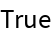#Function Repository Resource:

# CorrespondingIntegers

Create a list of positive integers containing as many elements as the expression to which it is applied

Contributed by: Seth J. Chandler
 ResourceFunction["CorrespondingIntegers"][expr] gives a list {1…n} of positive integers where n is the length of expr. ResourceFunction["CorrespondingIntegers"][expr,start,jump] gives a list {start,start+jump,…,start+(n-1)×jump} where n is the length of expr. ResourceFunction["CorrespondingIntegers"][start,jump] creates an operator that, when applied to an expression expr, gives a list {start,start+jump,…,start+(n-1)×jump} where n is the length of expr.

## Examples

### Basic Examples

Create a list of the positive integers corresponding to letters “a” to “j”:

 In:=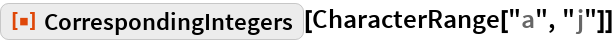Out=Create a list of integers starting with 5 and incrementing by 2 each time that correspond to the letters "a" to "j":

 In:=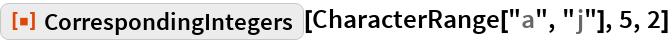Out=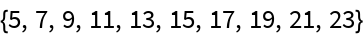Create an operator which, when it an expression, creates a list with the same number of parts as that expression, in which the first element is 1, and in which each successive value is 1 higher:

 In:=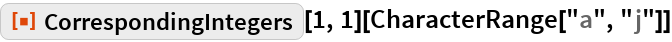Out=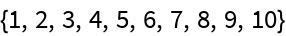### Scope

The function works on expressions that are not lists :

 In:=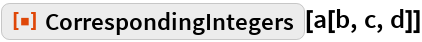Out=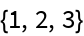Because the length of an Association is equal to its number of key value pairs, the function can be sensibly applied to associations:

 In:=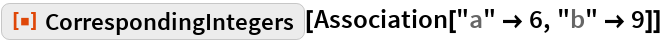Out=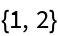The function looks only at the first level of an expression to determine the correspondence :

 In:=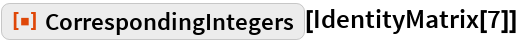Out=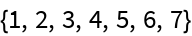Using non - integer values for the starting value and/or the jump will give a warning, as this is not the purpose for which the function is intended: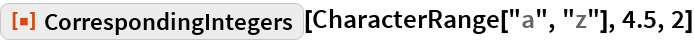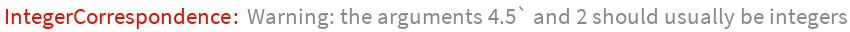Out=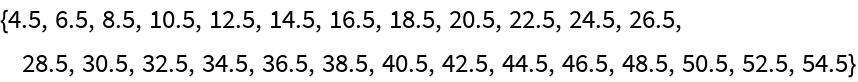### Applications

Use CorrespondingIntegers to develop indices into a training and test set used for machine learning:

 In:=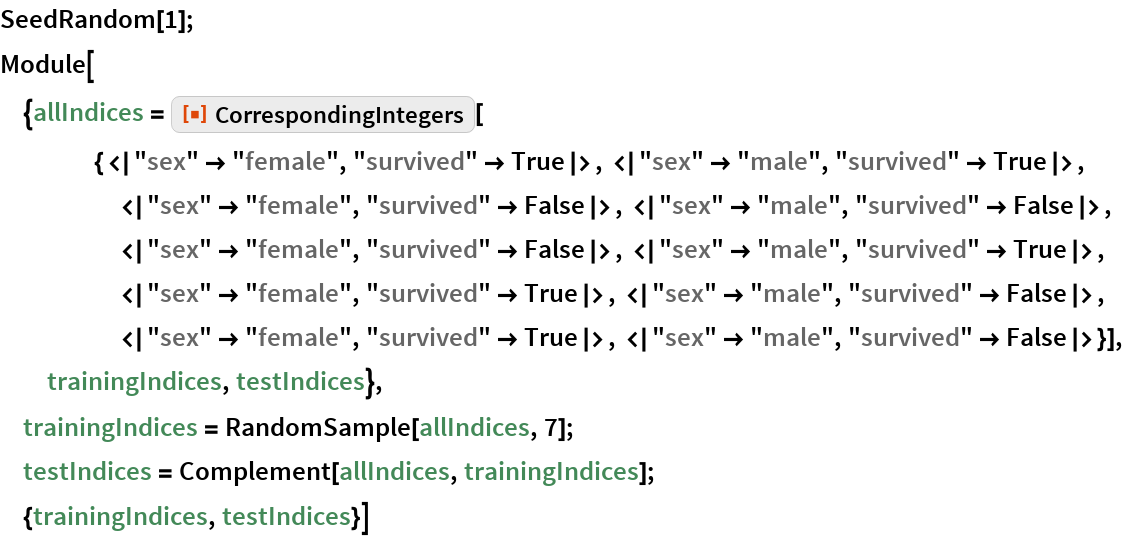Out=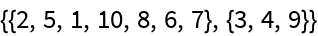Index the terms in some document both backward and forward:

 In:=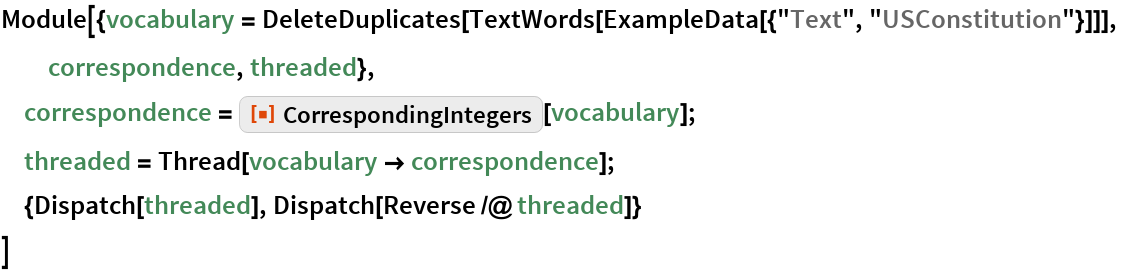Out=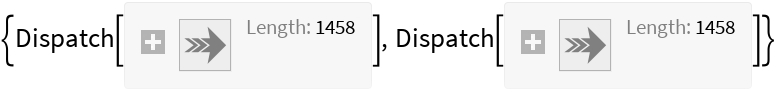### Properties and Relations

This function can be combined with Thread to emulate the action of MapIndexed at level 1 where one takes the first part of the index:

 In:=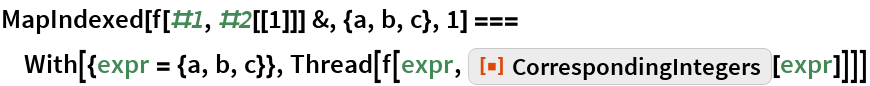Out=